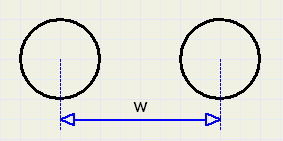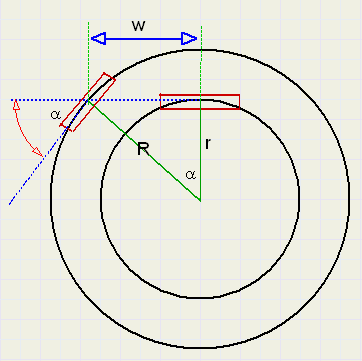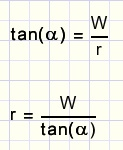Turning Radius calculationIntroduction
This radius depends on two things:
- the wheelbase w , which is the distance between the front- and the rear wheel
- the angle a of the front wheel
We suppose that only the front wheel is able to turn.
See figure below:side view top view

Calculation
The front and rear wheel follow a circle with the same center.
At all times, the direction is perpendicular to the radius.
See figure below:movement

The radius of the front wheel is R, the rear wheel r.
From the figure above we conclude:also